Скачать презентацию TODAY S OUTCOMES SUN AND MOON — Review first

• Количество слайдов: 6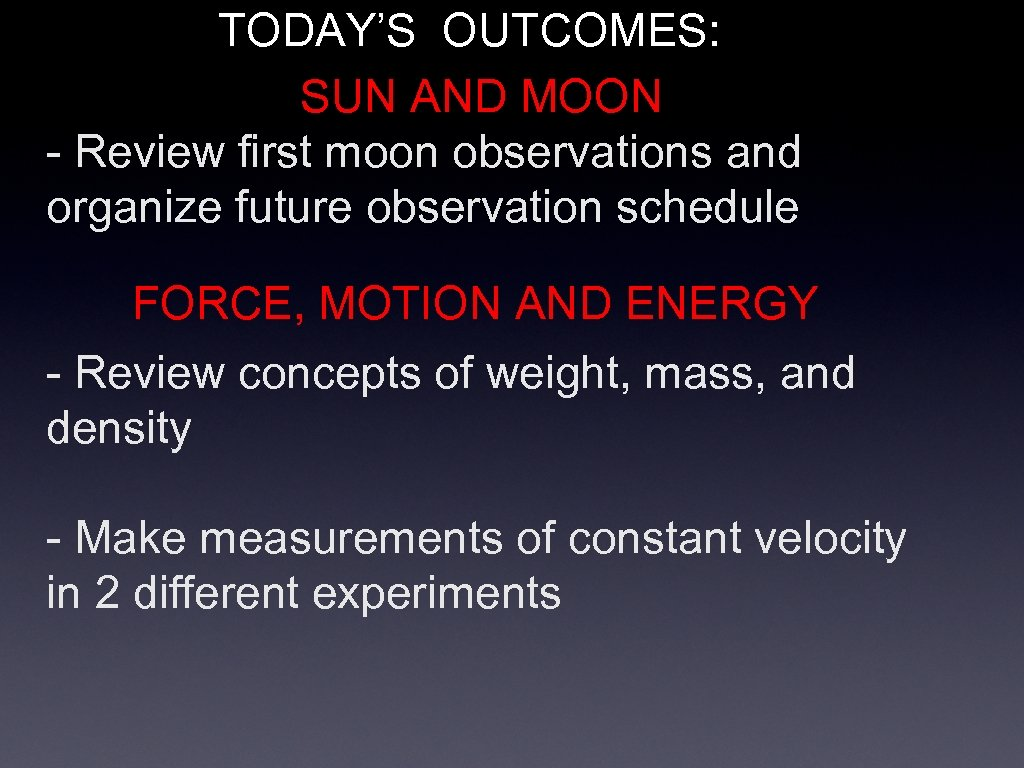TODAY’S OUTCOMES: SUN AND MOON - Review first moon observations and organize future observation schedule FORCE, MOTION AND ENERGY - Review concepts of weight, mass, and density - Make measurements of constant velocity in 2 different experiments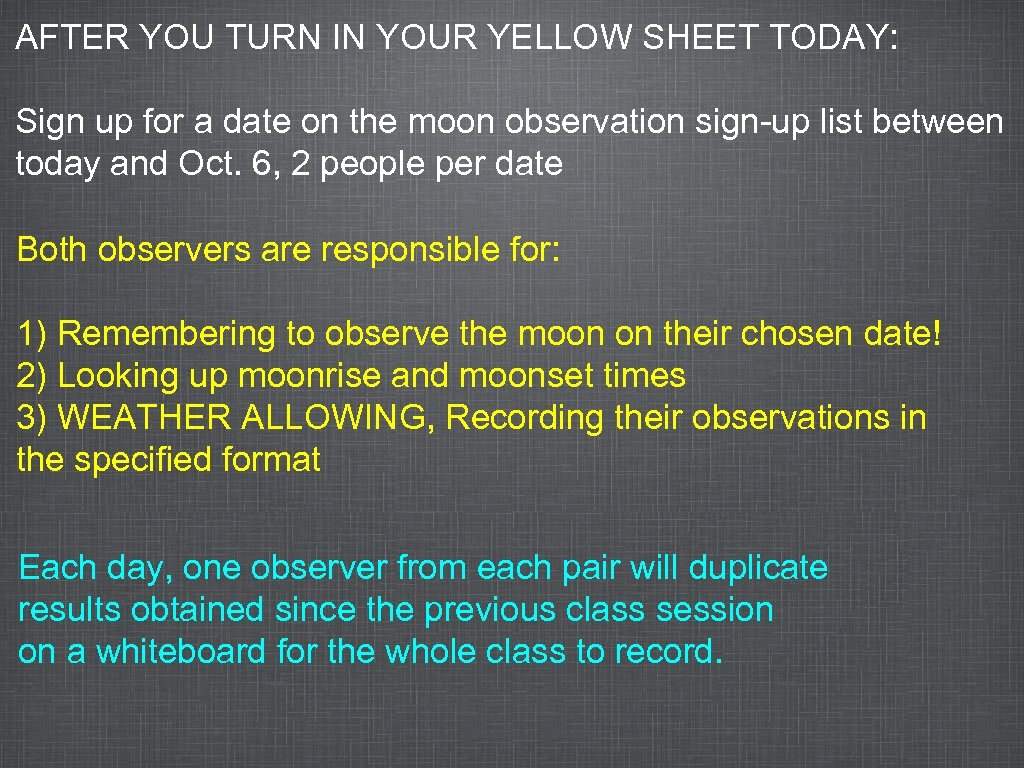AFTER YOU TURN IN YOUR YELLOW SHEET TODAY: Sign up for a date on the moon observation sign-up list between today and Oct. 6, 2 people per date Both observers are responsible for: 1) Remembering to observe the moon on their chosen date! 2) Looking up moonrise and moonset times 3) WEATHER ALLOWING, Recording their observations in the specified format Each day, one observer from each pair will duplicate results obtained since the previous class session on a whiteboard for the whole class to record.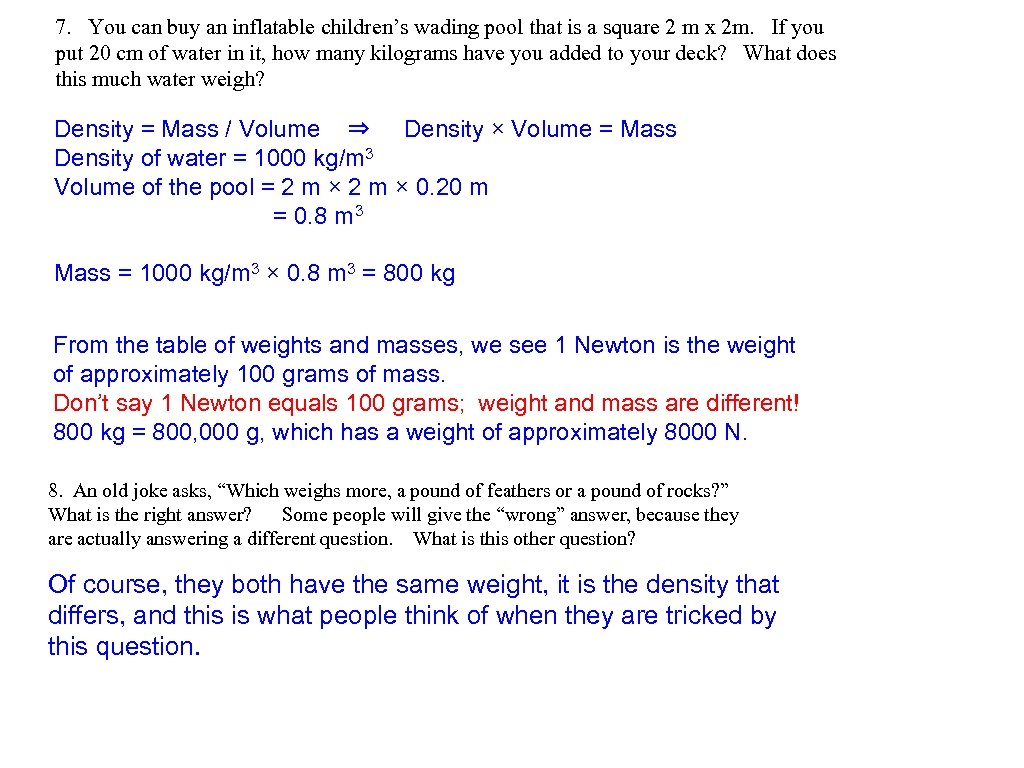7. You can buy an inflatable children’s wading pool that is a square 2 m x 2 m. If you put 20 cm of water in it, how many kilograms have you added to your deck? What does this much water weigh? Density = Mass / Volume ⇒ Density × Volume = Mass Density of water = 1000 kg/m 3 Volume of the pool = 2 m × 0. 20 m = 0. 8 m 3 Mass = 1000 kg/m 3 × 0. 8 m 3 = 800 kg From the table of weights and masses, we see 1 Newton is the weight of approximately 100 grams of mass. Don’t say 1 Newton equals 100 grams; weight and mass are different! 800 kg = 800, 000 g, which has a weight of approximately 8000 N. 8. An old joke asks, “Which weighs more, a pound of feathers or a pound of rocks? ” What is the right answer? Some people will give the “wrong” answer, because they are actually answering a different question. What is this other question? Of course, they both have the same weight, it is the density that differs, and this is what people think of when they are tricked by this question.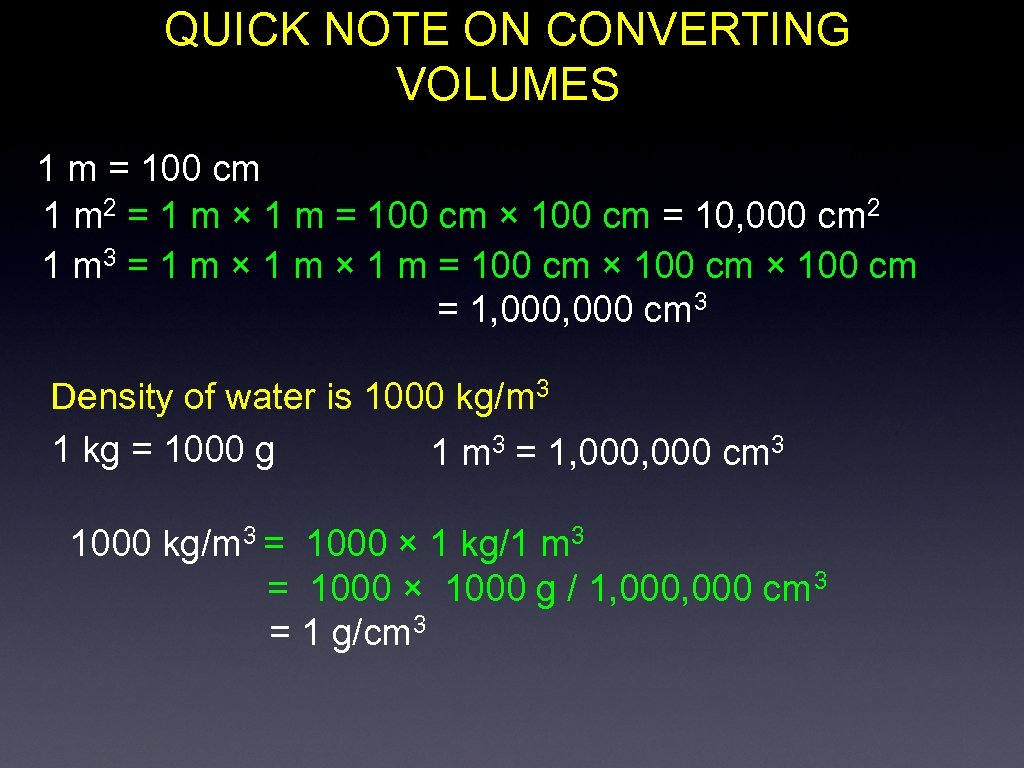QUICK NOTE ON CONVERTING VOLUMES 1 m = 100 cm 1 m 2 = 1 m × 1 m = 100 cm × 100 cm = 10, 000 cm 2 1 m 3 = 1 m × 1 m = 100 cm × 100 cm = 1, 000 cm 3 Density of water is 1000 kg/m 3 1 kg = 1000 g 1 m 3 = 1, 000 cm 3 1000 kg/m 3 = 1000 × 1 kg/1 m 3 = 1000 × 1000 g / 1, 000 cm 3 = 1 g/cm 3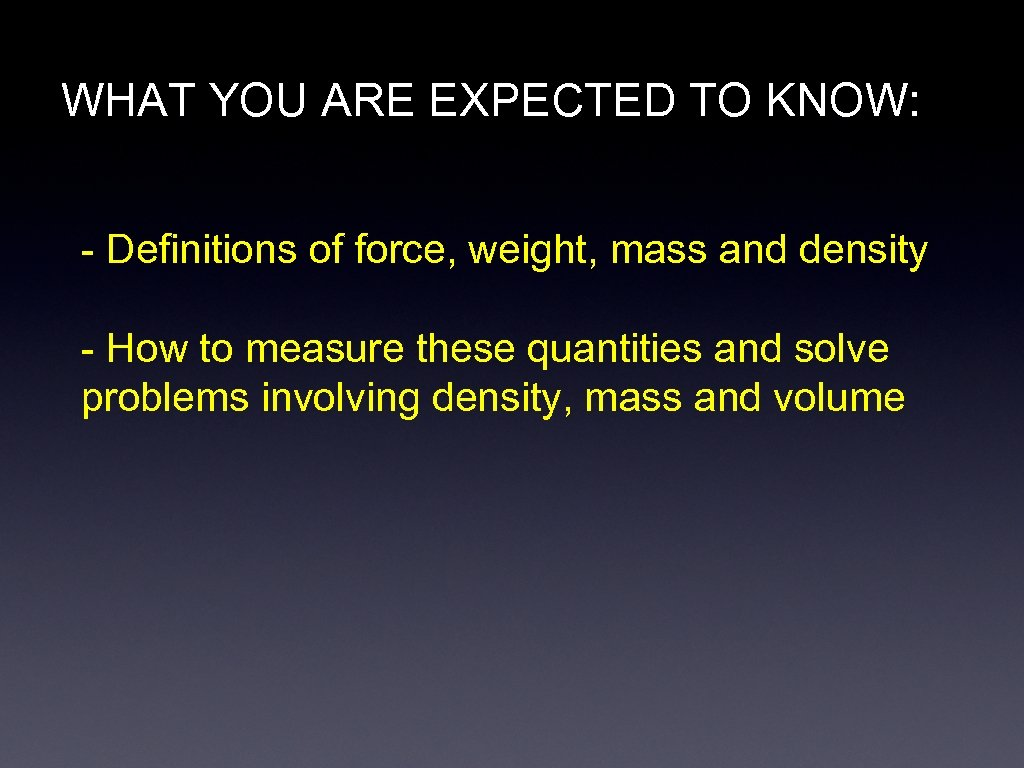WHAT YOU ARE EXPECTED TO KNOW: - Definitions of force, weight, mass and density - How to measure these quantities and solve problems involving density, mass and volume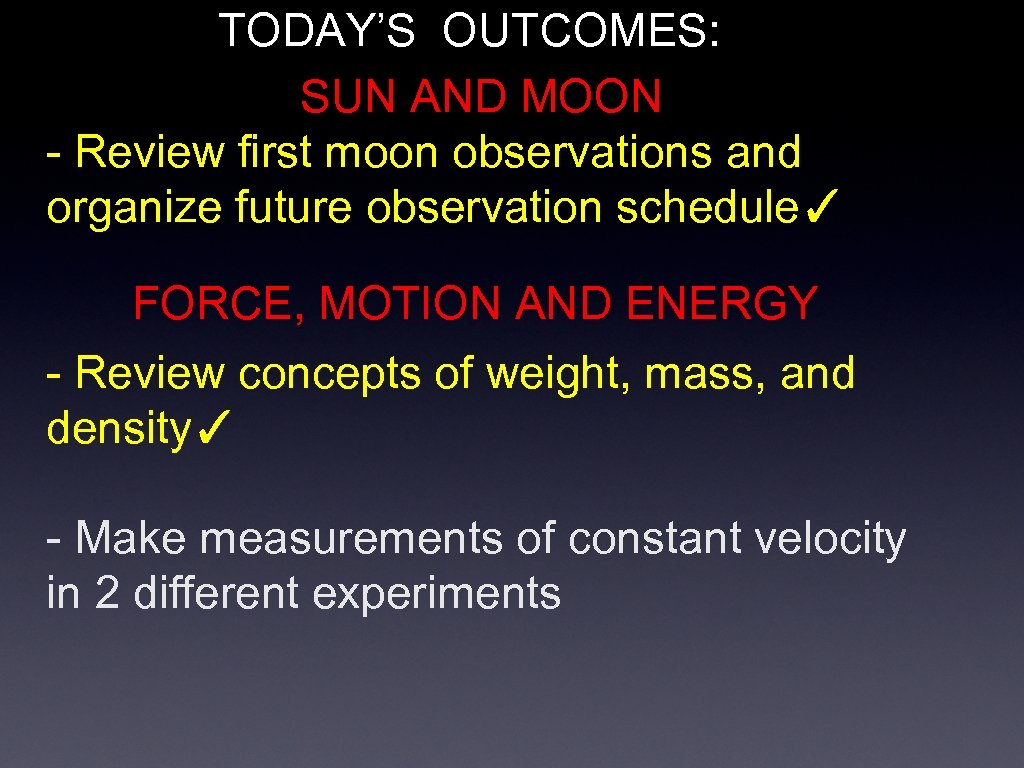TODAY’S OUTCOMES: SUN AND MOON - Review first moon observations and organize future observation schedule✓ FORCE, MOTION AND ENERGY - Review concepts of weight, mass, and density✓ - Make measurements of constant velocity in 2 different experiments